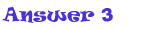To find out, multiply 1.45 by 2 then !.15 by 3, then 1.90 by 2, add 2.90 by 3.45 and 3.88. Lastly subtract 20 by 10.15(as shown below):

1.45 X 2 = 2.90

1.15 X 3 = 3.45

1.90 X 2 = 3.80

2.90 + 3.45 +3.80 = 10.15

20 - 10.15 = 9.85

Isabel should get \$9.85 back.